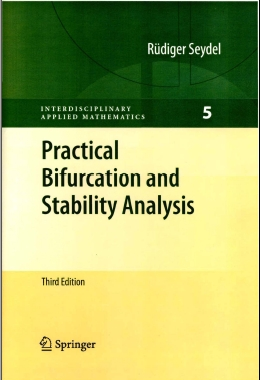# Contentscontents as PDF
index as PDF

1. Introduction and Prerequisites basics of ODEs, including equilibria, periodic solutions, stability, simulation; maps and stability of fixed points; fundamental numerical methods

2. Basic Nonlinear Phenomena bifurcations

3. Applications and Extensions delay differential equations; nonsmooth dynamics; DAEs; nerve impulses; waves and pattern formation; deterministic risk

4. Principles of Continuation homotopy, predictor-corrector methods, step control

5. Calculation of the Branching Behavior of Nonlinear Equations calculating stability; bifurcation test functions; calculating bifurcation points; branch switching

6. Calculating Branching Behavior of Boundary-Value Problems numerical bifurcation for ODE boundary-value problems

7. Stability of Periodic Solutions monodromy matrix, Poincare map; period doubling; calculating bifurcation behavior

8. Qualitative Instruments normal forms; elementary catastrophes; center manifolds

9. Chaos attractors; routes to chaos; fractal dimension; control of chaos; Liapunov exponents; power spectra

Appendices basic background material

Dec'09
seydel "at" math.uni-koeln.de